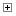##Classes

Defined in: qdt.js.

Class Summary
Constructor Attributes Constructor Name and Description

Field Summary
Field Attributes Field Name and Description

The maximum number of points stored in a quad tree node before it is subdivided.

In a subdivided quad tree this represents the top right subtree.

In a subdivided quad tree this represents the top left subtree.

Point storage.

In a subdivided quad tree this represents the bottom right subtree.

In a subdivided quad tree this represents the bottom left subtree.
Class Detail
Parameters:
{Array} bbox
Bounding box of the new quad (sub)tree.

Field Detail
capacity
The maximum number of points stored in a quad tree node before it is subdivided.
Default Value:
10

northEast
In a subdivided quad tree this represents the top right subtree.

northWest
In a subdivided quad tree this represents the top left subtree.

points
Point storage.

southEast
In a subdivided quad tree this represents the bottom right subtree.

southWest
In a subdivided quad tree this represents the bottom left subtree.

Documentation generated by JsDoc Toolkit 2.4.0 on Tue Mar 23 2021 15:17:42 GMT+0100 (Mitteleuropäische Normalzeit)